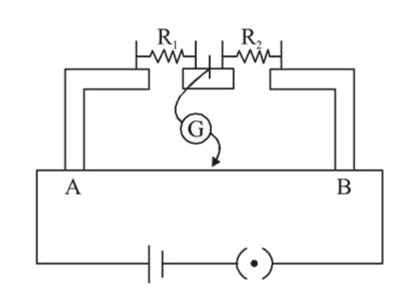# In the experimental set up of metre bridge shown in the figure,Question:

In the experimental set up of metre bridge shown in the figure, the null point is obtaine data distance of $40 \mathrm{~cm}$ from A. If a $10 \Omega$ resistor is connected in series with $\mathrm{R}_{1}$, the null point shifts by $10 \mathrm{~cm}$. The resistance that should be connected in parallel with $\left(\mathrm{R}_{1}+10\right) \Omega$ such that the null point shifts back to its initial position is :1. (1) $20 \Omega$

2. (2) $40 \Omega$

3. (3) $60 \Omega$

4. (4) $30 \Omega$

Correct Option: , 3

Solution:

(3) Initially at null deflection $\frac{R_{1}}{R_{2}}=\frac{2}{3}$           ...(1)

Finally at null deflection, when null point is shifted

$\frac{\mathrm{R}_{1}+10}{\mathrm{R}_{2}}=1 \Rightarrow \mathrm{R}_{1}+10=\mathrm{R}_{2} \ldots$

Solving equations (i) and (ii) we get

$\frac{2 R_{2}}{3}+10=R_{2}$

$10=\frac{R_{2}}{3} \Rightarrow R_{2}=30 \Omega$

$\& \mathrm{R}_{1}=20 \Omega$

Now if required resistance is $\mathrm{R}$ then

$\frac{\frac{30 \times R}{30+R}}{30}=\frac{2}{3}$

$R=60 \Omega$